## 【Terraform】快速实践"Hello World"

### 一、环境准备

Terraform 和 Docker

`Provider`插件缓存配置

`Terraform`的整个执行流程中，我们在使用到`Terraform init`命令时，该工具会分析我们代码中使用到的`Provider`，并尝试下载`Provider`插件到本地，如果我们不进行特殊的设置，每次执行`init`都会尝试去下载插件，并且每个项目都回保存自己独立的插件，相当浪费空间。这怎么办呢？

``````#务必确保这个目录下已经创建好plugin-cache这个文件夹
export TF_PLUGIN_CACHE_DIR="\$HOME/.terraform.d/plugin-cache"

``````plugin_cache_dir = "\$HOME/.terraform.d/plugin-cache"

### 二、实战

#### 2.1 定义资源

[terraform-provider-docker​]  github.com/kreuzwerker…

``````terraform {
#定义所需的Provider
required_providers {
docker = {
source  = "kreuzwerker/docker"
version = "~> 2.13.0"
}
}
}
​
# 配置docker provider
provider "docker" {}
​
#创建一个docker镜像资源
resource "docker_image" "nginx" {
name         = "nginx:latest"
keep_locally = false
}
#创建一个docker容器资源
#这部分的资源定义，类似"docker run --name nginx -p8080:80 -d nginx:latest"
resource "docker_container" "nginx" {
image = docker_image.nginx.latest
name  = "tutorial"
ports {
internal = 80
external = 8000
}
}
#接下来还可以继续定义服务资源

#### 2.2 执行创建

``````#创建目录
\$mkdir learn-terraform-docker-container
#进入目录
\$cd learn-terraform-docker-container

``````\$Terraform init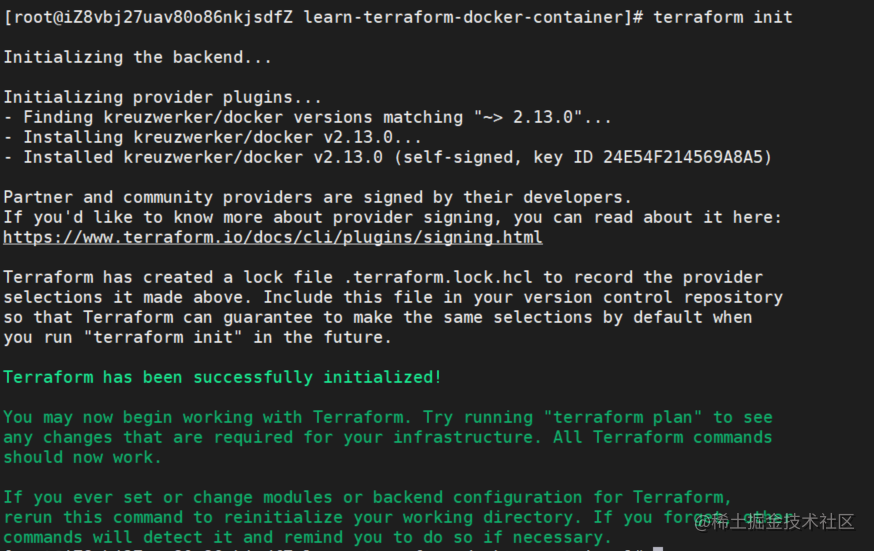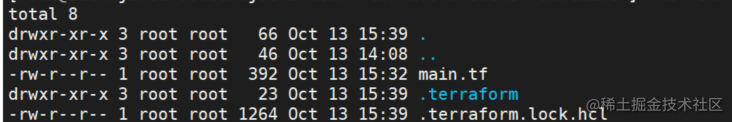`.terraform`隐藏文件夹中存储着我们下载的`Provider`插件内容，`.terraform.lock.hcl`记录了`provider`插件的一些依赖信息。如果进入我们前面设置的缓存插件目录，会多了这个插件的内容。

``````\$terraform plan

``````Terraform used the selected providers to generate the following execution plan. Resource actions are indicated with the following symbols:
+ create
​
Terraform will perform the following actions:
​
# docker_container.nginx will be created
+ resource "docker_container" "nginx" {
+ attach           = false
+ bridge           = (known after apply)
+ command          = (known after apply)
+ container_logs   = (known after apply)
+ entrypoint       = (known after apply)
+ env              = (known after apply)
+ exit_code        = (known after apply)
+ gateway          = (known after apply)
+ hostname         = (known after apply)
+ id               = (known after apply)
+ image            = (known after apply)
+ init             = (known after apply)
+ ip_address       = (known after apply)
+ ip_prefix_length = (known after apply)
+ ipc_mode         = (known after apply)
+ log_driver       = "json-file"
+ logs             = false
+ must_run         = true
+ name             = "tutorial"
+ network_data     = (known after apply)
+ remove_volumes   = true
+ restart          = "no"
+ rm               = false
+ security_opts    = (known after apply)
+ shm_size         = (known after apply)
+ start            = true
+ stdin_open       = false
+ tty              = false
​
+ healthcheck {
+ interval     = (known after apply)
+ retries      = (known after apply)
+ start_period = (known after apply)
+ test         = (known after apply)
+ timeout      = (known after apply)
}
​
+ labels {
+ label = (known after apply)
+ value = (known after apply)
}
​
+ ports {
+ external = 8000
+ internal = 80
+ ip       = "0.0.0.0"
+ protocol = "tcp"
}
}
​
# docker_image.nginx will be created
+ resource "docker_image" "nginx" {
+ id           = (known after apply)
+ keep_locally = false
+ latest       = (known after apply)
+ name         = "nginx:latest"
+ output       = (known after apply)
+ repo_digest  = (known after apply)
}
​
Plan: 2 to add, 0 to change, 0 to destroy.

``````\$terraform apply

``````Terraform used the selected providers to generate the following execution plan. Resource actions are indicated with the following symbols:
+ create
​
Terraform will perform the following actions:
​
# docker_container.nginx will be created
+ resource "docker_container" "nginx" {
+ attach           = false
+ bridge           = (known after apply)
+ command          = (known after apply)
+ container_logs   = (known after apply)
+ entrypoint       = (known after apply)
+ env              = (known after apply)
+ exit_code        = (known after apply)
+ gateway          = (known after apply)
+ hostname         = (known after apply)
+ id               = (known after apply)
+ image            = (known after apply)
+ init             = (known after apply)
+ ip_address       = (known after apply)
+ ip_prefix_length = (known after apply)
+ ipc_mode         = (known after apply)
+ log_driver       = "json-file"
+ logs             = false
+ must_run         = true
+ name             = "tutorial"
+ network_data     = (known after apply)
+ remove_volumes   = true
+ restart          = "no"
+ rm               = false
+ security_opts    = (known after apply)
+ shm_size         = (known after apply)
+ start            = true
+ stdin_open       = false
+ tty              = false
​
+ healthcheck {
+ interval     = (known after apply)
+ retries      = (known after apply)
+ start_period = (known after apply)
+ test         = (known after apply)
+ timeout      = (known after apply)
}
​
+ labels {
+ label = (known after apply)
+ value = (known after apply)
}
​
+ ports {
+ external = 8000
+ internal = 80
+ ip       = "0.0.0.0"
+ protocol = "tcp"
}
}
​
# docker_image.nginx will be created
+ resource "docker_image" "nginx" {
+ id           = (known after apply)
+ keep_locally = false
+ latest       = (known after apply)
+ name         = "nginx:latest"
+ output       = (known after apply)
+ repo_digest  = (known after apply)
}
​
Plan: 2 to add, 0 to change, 0 to destroy.
​
Do you want to perform these actions?
Terraform will perform the actions described above.
Only 'yes' will be accepted to approve.
​
Enter a value:yes
​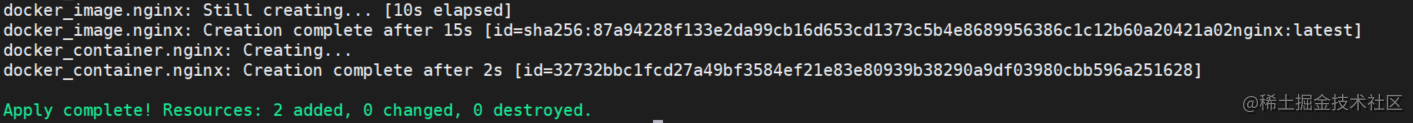``````\$docker ps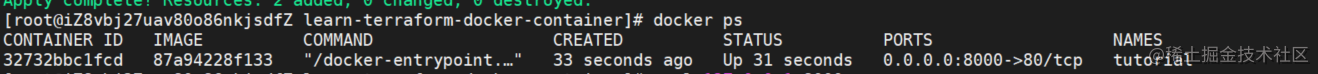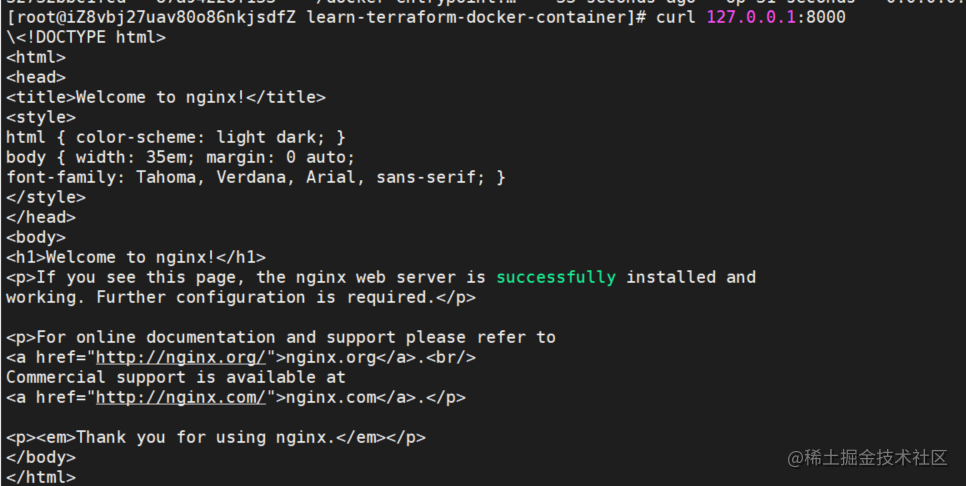### 2.3 执行销毁

``````\$terraform destroy